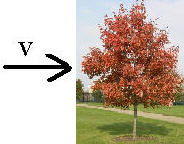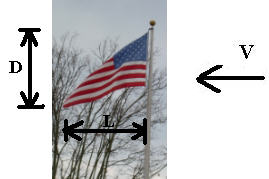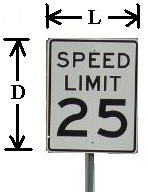Drag Force Calculation - enter Drag Coefficient

Compute Drag Force, Velocity, or Area

Register to enable "Calculate" button.

 Select fluid: Air, 20C, 1 atm CO2, 20C, 1 atm Methane, 20C, 1 atm Water, 20C (68F) Water, 4C (39F) Seawater, 16C (60F) SAE 30 oil, 16C (60F) Gasoline, 16C (60F) Another fluid Select calculation: Compute Reference Area Compute Velocity Compute Force Fluid Density: g/cm3 kg/m3 N/m3 slug/ft3 lb/ft3 Drag Coefficient, C: Reference Area, A: cm2 m2 inch2 ft2 Velocity, V: m/s km/hr ft/s mile/hr (mph) nautical mile/hr (knot) Force, F: dyne Newton pound ©2014 LMNO Engineering, Research, and Software, Ltd. http://www.LMNOeng.com

Units in Drag Force calculator: cm=centimeter, ft=feet, g=gram, hr=hour, kg=kilogram, km=kilometer, lb=pound, m=meter, N=Newton, s=second

Introduction
Drag force is caused by a fluid (such as water or air; or any liquid or gas) impinging upon an object. The drag force is a function of the fluid velocity and density along with the object's reference area and drag coefficient. The drag coefficient may further be a function of the Reynolds number. Reynolds number depends on the fluid density, viscosity, and velocity as well as the object's characteristic length.

Drag Force Equation (Blevins, 2003 and Munson et al., 1998 and others)

F = 0.5 C ρ A V2

Notation
Our calculation allows you to use a variety of units with all of the conversions completed internally. The units below are consistent units for the drag force equation.
A = Reference area as (see figures below), m2.
C = Drag coefficient (see figures below), unitless.
F = Drag force, N.
V = Velocity, m/s.
ρ = Density of fluid (liquid or gas), kg/m3.

Drag coefficients for various objects (photographs taken by LMNO Engineering).

 Tree (C values from Munson et al., 1998) A=Tree frontal area C=0.43 if V=10 m/s (36.0 km/h, 22.4 mph) C=0.26 if V=20 m/s (72.0 km/h, 44.7 mph) C=0.20 if V=30 m/s (108 km/h, 67.1 mph)Flag (C values from Munson et al., 1998) A=DL C=0.07 if L/D=1 C=0.12 if L/D=2 C=0.15 if L/D=3Thin Rectangular Plate (C from Blevins, 2003) Flow coming at the sign A=DL C=1.05 if L/D=1.0 C=1.10 if L/D=2.0 C=1.12 if L/D=4.0 C=1.20 if L/D=8.0 C=1.22 if L/D=10.0 C=1.22 if L/D=12.0 C=1.33 if L/D=17.8 C=1.90 if L/D=infinityMessages
"Need Density > 0", "Need C > 0", "Need Area > 0", "Need Velocity > 0", "Need Force > 0".

References
Blevins, Robert D. 2003. Applied Fluid Dynamics Handbook. Krieger Publishing Co.

Munson, Bruce R., Donald F. Young, and Theodore H. Okiishi. 1998. Fundamentals of Fluid Mechanics. John Wiley and Sons, Inc. 3ed.

LMNO Engineering, Research, and Software, Ltd.
7860 Angel Ridge Rd.   Athens, Ohio 45701  USA   Phone: (740) 592-1890
LMNO@LMNOeng.com    http://www.LMNOeng.com

To: